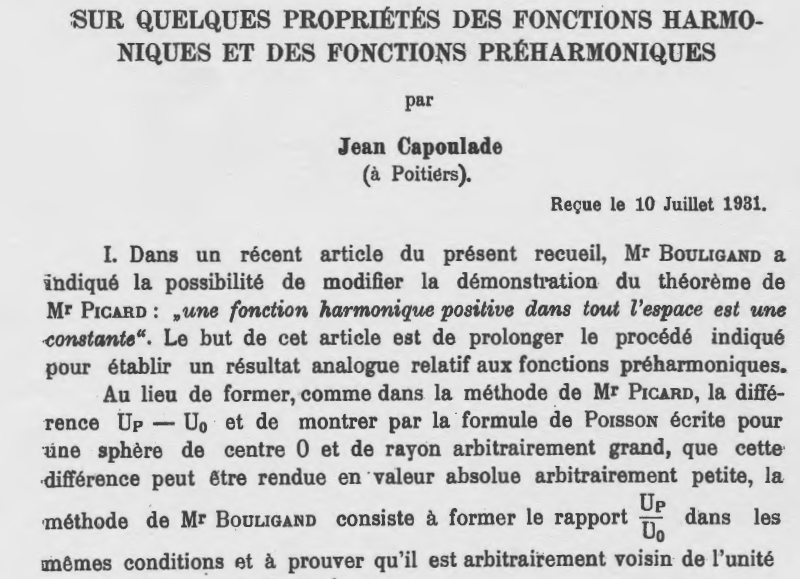# The origin of Discrete `Liouville's theorem'

It is known that discrete Liouville's theorem for harmonic functions on $\mathbb{Z}^2$ was proved by Heilbronn (On discrete harmonic functions. - Proc. Camb. Philos. Soc. , 1949, 45, 194-206).

If a bounded function $f : \mathbb Z^2 \rightarrow \mathbb{R}$ satisfies the following condition $$\forall (x, y) \in \mathbb{Z}^2,\quad f(x,y) = \dfrac{f(x + 1, y)+f(x, y + 1) + f(x - 1, y) +f(x, y - 1)}{4}$$ then f is constant function.

(A stronger version of discrete “Liouville's theorem” also follows from Heilbronn's proof.)

But recently I knew from Alexander Khrabrov that there is an older article of Capoulade with almost the same result (Sur quelques proprietes des fonctions harmoniques et des fonctions preharmoniques, - Mathematica (Cluj), 6 (1932), 146-151.)

Unfortunately the last article is not available for me. Does anybody have an access to this paper? Is this really the first proof discrete Liouville's theorem?

(This question is inspired by Liouville Theorem from Mathematics)

• I'll make a trip to Leiden University library today or tomorrow to obtain this elusive article by Capoulade (I hope). Dec 28, 2013 at 13:13
• no luck yet, I know it's out there in storage, and I'm confident I'll get it eventually, but if there's someone with easier access --- don't let me hold you back! Dec 30, 2013 at 7:36
• got it (first time this journal left it's offsite storage...); I'll scan it and post it as a "partial answer" shortly. Dec 31, 2013 at 11:00Mathematica (Cluj), 6, 146-151 (1932)

In a recent article from this journal, Mr. Bouligand has indicated the possibility of a modified proof of a theorem of Mr. Picard: "a harmonic function that is positive in the whole space is a constant". The purpose of this article is to continue along the same lines to prove an analogous result for preharmonic functions.

So this seems to be the required theorem. If help is needed with the French text of the paper, let me know.

• Dear Carlo, thank you very much for the article. It is at least a half of an answer. Probably Capoulade was the first who had proved this theorem. Dec 31, 2013 at 12:34
• If I understood correctly, one of Capoulade's proofs is based on a result by La Roux, who gave explicit formula for the solution of Dirichlet problem in rectangle. The last article is available in Gallica, see portail.mathdoc.fr/JMPA/… Dec 31, 2013 at 12:52
• Rather off-topic, but I wonder whether it is possible to prove Liouville's theorem considering a function like $F(x,y)=\sum_{i,j} f(i,j) x^iy^j$. (As written, this does not make much sence, of course, since the series diverges.) We then have $$4F(x,y) = \sum_{i,j} \left(xf(i-1,j)x^{i-1}j+\dotsb+\frac1yf(i,j+1)x^iy^{j+1} \right)$$ whence $4F(x,y)=(x+1/x+y+1/y)F(x,y)$, implying $F(x,y)=0$.
– Seva
Dec 13, 2019 at 9:53